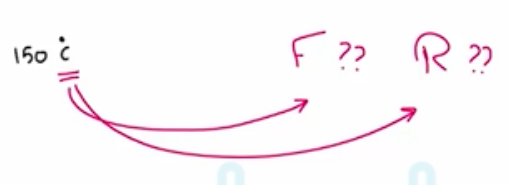Need Help?

Subscribe to Thermodynamics

###### \${selected_topic_name}
• Notes

$\text { What is the temperature of the heated air at } 150^{\circ} \mathrm{C} \text { in }^{\circ} \mathrm{F} \text { and } \mathrm{R} ?$$T(^{\circ}{F})=1.8 T(c^{\circ})+32=1.8(150)+32=302 \ ^{\circ} \mathrm{f}$

$T(R)=T(^{\circ}F)+460=302+460=762 R$

$\begin{array}{l}{\text { The temperature of a system rises by } 45^{\circ} \mathrm{C} \text { during a heating process. }} \\ {\text { Express this rise in temperature in kelvins. }}\end{array}$

$\Delta T(k)=\Delta T(^\circ{c})$

$45 k=45^{\circ} \mathrm{C}$

$\Delta { F: 10F }$

$\Delta T=\frac{10}{1.8}=5.6 \ ^\circ{c}$

$\Delta T=\frac{10}{1.8}=5.6 k$

$\Delta T=10 \ ^{\circ} F=10 R$